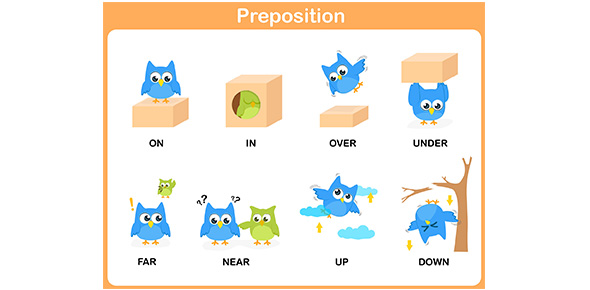# Tplc Then Vs Than Quiz Questions

Approved & Edited by ProProfs Editorial Team
The editorial team at ProProfs Quizzes consists of a select group of subject experts, trivia writers, and quiz masters who have authored over 10,000 quizzes taken by more than 100 million users. This team includes our in-house seasoned quiz moderators and subject matter experts. Our editorial experts, spread across the world, are rigorously trained using our comprehensive guidelines to ensure that you receive the highest quality quizzes.
| By Charliemahoney
C
Charliemahoney
Community Contributor
Quizzes Created: 3 | Total Attempts: 7,909
Questions: 10 | Attempts: 1,830SettingsMost of the time you will find many people confusing the use of the words then and than in constructing and joining sentences. What do you think is the difference? Supplement your knowledge below.

• 1.

### My jack-o-lantern is far better (then,  than) any other jack-o-lantern.

• A.

Then

• B.

Than

B. Than
Explanation
The correct answer is "than". In this sentence, "than" is used to compare the speaker's jack-o-lantern to other jack-o-lanterns. It indicates that the speaker's jack-o-lantern is superior or of higher quality compared to the others. "Then" is not the correct choice because it is used to indicate a sequence of events or a time frame, which is not relevant in this context.

Rate this question:

• 2.

### First, we study the homework; (then,  than) we take the test.

• A.

Then

• B.

Than

A. Then
Explanation
The word "then" is the correct answer because it indicates the correct sequence of events. In this sentence, it is stating that studying the homework comes before taking the test. "Than" is used to make comparisons and would not be appropriate in this context.

Rate this question:

• 3.

### If you come with me, (then,  than) we will go to the circus.

• A.

Then

• B.

Than

A. Then
Explanation
The correct answer is "then" because it is used to indicate a sequence of events or actions. In this sentence, "then" is used to show that if the person comes with the speaker, the next action will be going to the circus. "Than" is used to make comparisons between two things and is not suitable in this context.

Rate this question:

• 4.

### This class scores higher (then,  than) any other class.

• A.

Then

• B.

Than

B. Than
Explanation
The word "than" is the correct answer because it is used to compare two things or groups. In this sentence, it is comparing the class being referred to with other classes. The word "then" is used to indicate a sequence of events or a consequence, which does not fit the context of the sentence.

Rate this question:

• 5.

### He leaped on the thief, tied him up, and (then,  than) called the police.

• A.

Then

• B.

Than

A. Then
Explanation
In this sentence, "then" is the correct word choice. The word "then" is used to indicate the order of events, showing that after the speaker tied up the thief, they proceeded to call the police. On the other hand, "than" is used for making comparisons, which is not appropriate in this context.

Rate this question:

• 6.

### This test seems to be easier (then,  than) the last one.

• A.

Then

• B.

Than

B. Than
Explanation
The correct answer is "than" because it is used to make a comparison between two things. In this sentence, the speaker is comparing the current test to the last one and stating that the current test is easier.

Rate this question:

• 7.

### The discussion lasted much longer (than,   then) we thought it would.

• A.

Then

• B.

Than

B. Than
Explanation
The correct answer is "than". In this sentence, "than" is used to compare the duration of the discussion with our initial expectation. The word "then" refers to a specific time or sequence of events, which does not make sense in this context.

Rate this question:

• 8.

### That cat is much bigger (then,  than) that dog.

• A.

Then

• B.

Than

B. Than
Explanation
The word "than" is the correct answer because it is used to make a comparison between the size of the cat and the dog. In this sentence, we are comparing the size of the cat to the size of the dog and stating that the cat is bigger. "Than" is the appropriate word to use in this context to indicate the comparison.

Rate this question:

• 9.

### His aroma is more (then,  than) I can stand.

• A.

Then

• B.

Than

B. Than
Explanation
The correct answer is "than". In this sentence, "than" is used to compare the intensity of the aroma to the speaker's tolerance level. It indicates that the aroma is exceeding the speaker's ability to tolerate it. "Then" is not appropriate in this context as it is used to indicate a sequence of events or a consequence.

Rate this question:

• 10.

### The storm poured down, but (then,  than) it ended.

• A.

Then

• B.

Than

A. Then
Explanation
The word "then" is the correct answer in this case. It is used to indicate the sequence of events, suggesting that the storm poured down first and then it ended. On the other hand, "than" is used for making comparisons, which is not relevant in this sentence.

Rate this question:

Related TopicsBack to top